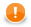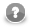### Iteration Statements

Iteration statements repeat some processes during which some inner `Statements` are executed repeatedly until the `Condition` that limits the execution cycle becomes false or they are executed for all values of the same data type.

#### For Loop

Firstly, the Initialization is set up. Secondly, the `Condition` is evaluated and if its value is true, the `Statement` is executed. Finally, the `Iteration` is made.

During the next cycle of the loop, the `Condition` is evaluated again and if it is true, `Statement` is executed and `Iteration` is made. This way the process repeats until the `Condition` becomes false. Then the loop is terminated and the process continues with the other part of the program.

If the `Condition` is false at the beginning, the process jumps over the `Statement` out of the loop.

• ```for (Initialization;Condition;Iteration)
Statement```
ImportantRemember that the `Initialization` part of the `For Loop` may also contain the declaration of the variable that is used in the loop. `Initialization`, `Condition` and `Iteration` are optional.

Example 65.21. For loop

```integer result = 1;
integer limit = 5;
for(integer i = 1; i <= limit; ++i) {
result = result * i;
}```

#### Do-While Loop

Firstly, the `Statement` is executed. Secondly, the value of the `Condition` is evaluated. If its value is true, the `Statement` is executed again and then the `Condition` is evaluated again and the loop either continues (if it is true again) or stops and jumps to the next or higher level subprocesses (if it is false).

Since the `Condition` is at the end of the loop, even if it is false at the beginning of the subprocess, the `Statement` is executed at least once.

• `do Statement while (Condition)`
```integer a = 5;
integer sum = 0;
do {
sum = sum + a;
a--;
} while (a > 3);```

#### While Loop

The processing depends on the value of the `Condition`. If its value is true, the `Statements` is executed and then the `Condition` is evaluated again and the processing either continues (if it is true again) or stops and jumps to the statement following the cycle (if it is false).

Since the `Condition` is at the beginning of the loop, if it is false before entrance to the loop, the `Statements` is not executed at all and the loop is jumped over.

• `while (Condition) Statement`
```integer a = 5;
integer sum = 0;
while ( a > 3 ) {
sum = sum + a;
a--;
```

#### For-Each Loop

The `foreach` statement is executed on all fields of the same data type within a container. Its syntax is as follows:

• `foreach (<data type> myVariable : iterableVariable) Statement`

All elements of the same data type (data type is declared in this statement) are searched in the `iterableVariable` container. The `iterableVariable` can be a list or a record. For each variable of the same data type, specified `Statement` is executed. It can be either a simple statement or a block of statements.

Thus, for example, the same `Statement` can be executed for all `string` fields of a record, etc.

NoteIt is possible to iterate over values of a map (i.e. not whole ``). Remember the type of the loop variable has to match the type of map's values: ``` map[string, integer] myMap; myMap["first"] = 1; myMap["second"] = 2; foreach(integer value: myMap) { printErr(value); // prints 1 and 2``` To obtain map's keys as a `list[]`, use the getKeys() function.